# Author Archive | Dr. Satnam Singh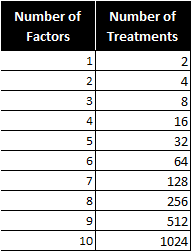## Fractional Factorial Designs with Minitab

What Are Fractional Factorial Experiments? In simple terms, a fractional factorial experiment is a subset of a full factorial experiment. Fractional factorials use fewer treatment combinations and runs. Fractional factorials are less able to determine effects because of fewer degrees of freedom available to evaluate higher order interactions. Fractional factorials can be used to screen [...]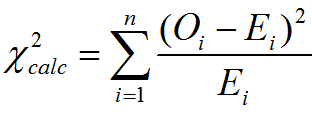## Chi Square Tests with Minitab

Chi Square (Contingency Tables) We have looked at hypothesis tests to analyze the proportion of one population vs. a specified value, and the proportions of two populations, but what do we do if we want to analyze more than two populations? A chi-square test is a hypothesis test in which the sampling distribution of the [...]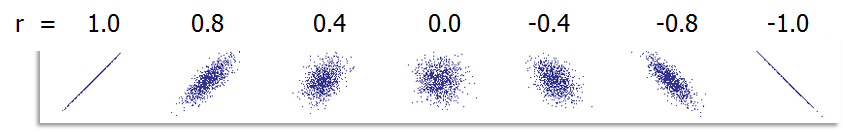## Correlation Coefficient with Minitab

Pearson’s Correlation Coefficient Pearson’s correlation coefficient is also called Pearson’s r or coefficient of correlation and Pearson’s product moment correlation coefficient (r), where r is a statistic measuring the linear relationship between two variables. What is Correlation? Correlation is a statistical technique that describes whether and how strongly two or more variables are related. Correlation [...]## One Sample Wilcoxon Test with Minitab

What is the One Sample Wilcoxon Test? The one sample Wilcoxon test is a hypothesis test to compare the median of one population with a specified value. Null Hypothesis (H0): η = η0 Alternative Hypothesis (Ha): η ≠ η0 It is an alternative test of one sample t-test when the distribution of the data is non-normal. It is [...]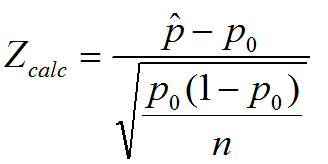## One Sample Proportion Test with Minitab

What is the One Sample Proportion Test? One sample proportion test is a hypothesis test to compare the proportion of one certain outcome (e.g. the number of successes per the number of trials, or the number of defects per the total number of opportunities) occurring in a population following the binomial distribution with a specified [...]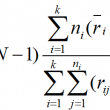## Kruskal Wallis Test with Minitab

Kruskal Wallis One-Way Analysis of Variance The Kruskal Wallis one-way analysis of variance is a statistical hypothesis test to compare the medians among more than two groups. Null Hypothesis (H0): η1 = η2 = … = ηk Alternative Hypothesis (Ha): at least one of the medians is different from others Where: ηi is the median [...]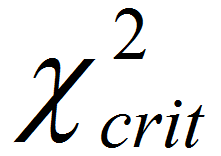## Median Test with Minitab

What is Mood’s Median Test? Mood’s median test is a statistical test to compare the medians of two or more populations. Null Hypothesis (H0): η1 = … = ηk Alternative Hypothesis (Ha): At least one of the medians is different from the others The symbol k is the number of groups of our interest and [...]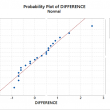## Paired t Test with Minitab

What is a Paired t Test? A Third type of a Two Sample t-Test is the Paired t Test.  This test is used when the two populations are dependent of each other, so each data point from one distribution corresponds to a data point in the other distribution. When using a paired t test, the [...]## Two Sample t Test with Minitab

What is Two Sample T Test? Two sample t test is a hypothesis test to study whether there is a statistically significant difference between the means of two populations. Null Hypothesis (H0): μ1 = μ2 Alternative Hypothesis Ha) : μ1 ≠ μ2 Where: μ1 is the mean of one population and μ2 is the mean of [...]